2020 UBS Quant Hackathon
Our Model, Results and Reflections from the 2020 UBS Quant Hackathon

Going into this competition, we had a 4-man team comprised of two seniors from Boston University and two sophomores (myself and a friend) from Cornell. We were competing against more than 300 teams of graduates and post-grads from across the world in two sub-events. In the first, we were tasked with building an algorithmic portfolio management strategy to outperform the S&P 500 on an annualized basis. In the second, our mission was to employ supervised learning models to predict the price of a derivative instrument. We split our team into two so that my friend from Cornell and I worked on the former event and the other half of the team worked on the latter. In this article, I will mainly discuss the first event including the problem that was presented, the model that we implemented, and results and reflections on our performance.

The Goal

The ultimate goal of this event was to develop a ‘payout function’, a function that would generate an equal-weighted long equities portfolio of 50 companies within the S&P 500 universe from 2007 to 2016. The data given to us included both daily OHLC (Open-High-Low-Close) price data for all companies within the available ticker universe and various economic data. Each time we wished to rebalance our portfolio, our net returns were penalized with a fraction of a basis point which acted as a sort of commission for our trades, so medium to long-term strategies were favored while high-frequency rebalancing strategies were discouraged. At any given point, companies could drop out of the S&P, forcing a rebalance. Our strategy would be considered invalidated if it contained ‘look-ahead bias’, meaning that on a date when our system chose to rebalance, it was not permitted to use any OHLC or economic data from any time period ahead. When backtested, our strategy must outperform the equal-weighted S&P 500 index which returned 5.31% per annum within the given time period and maintain a Shape Ratio above 0.60. In addition to returns, our portfolio would be judged on three metrics: information ratio, diversification, and robustness.

Our Model

Overview:

Given that this was our first time participating in a quant finance competition, we decided that our strategy would be somewhat simplistic. We chose to build a trend-following strategy in Python based on the Average Directional Movement Index (ADX) developed by J. Welles Wilder. The ADX returns a value between 0 and 100 where higher values are indicative of higher trend intensity, regardless of direction. When developing ADX, Wilder intended for it to be applied for commodities trading. However, at its core, ADX is a merely a gauge of trend strength, and it is calculated through OHLC data, a nearly-universal data form for securities in many market types, including equities. Maintaining the assumptions that commodities and equities are driven by similar supply-demand dynamics and that components of these dynamics, like trend-strength, can be decomposed from OHLC data and re-represented through technical indicators like ADX, it is reasonable to conclude that ADX can be applied to equities data to determine the highest momentum stocks. Thus, ADX (with slight modification) was chosen as the basis for our trend-following strategy.

Calculations: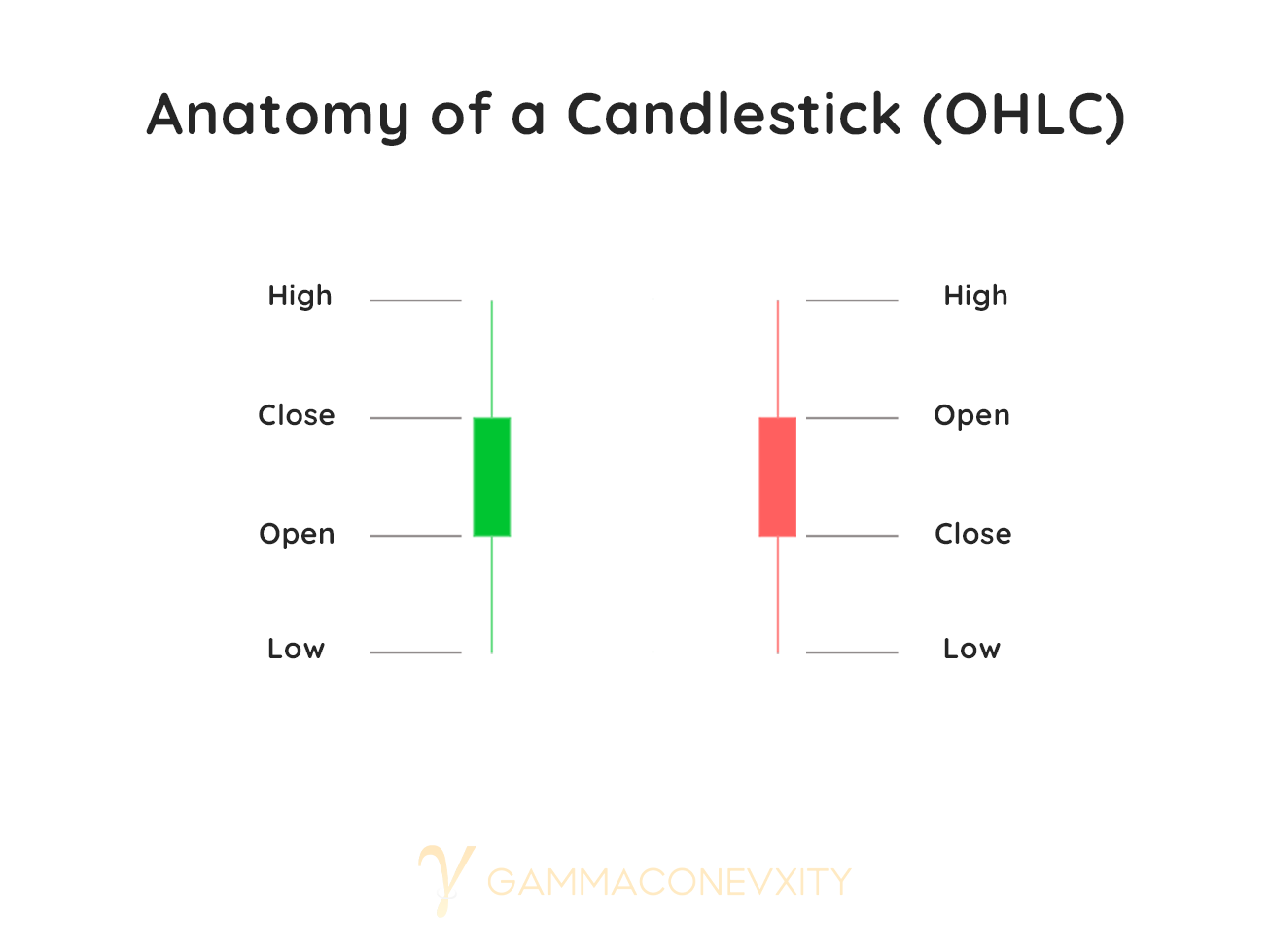Figure 1.1

Using OHLC price data (See Figure 1.1), we can derive ADX for a given date by first calculating three subcomponents:

1. Positive Directional Movement (+DM) and Negative Directional Movement (–DM)
2. True Range (TR)
3. Positive Directional Indicator (+DI) and Negative Directional Indicator (-DI)

1) The Positive Directional Movement (+DM) and Negative Directional Movement (-DM) define the largest portion of the current candle that is outside the range of the previous candle. They are calculated below (See figure 1.2) where t denotes the candle from the current time period, and t-1 denotes the candle from the previous time period.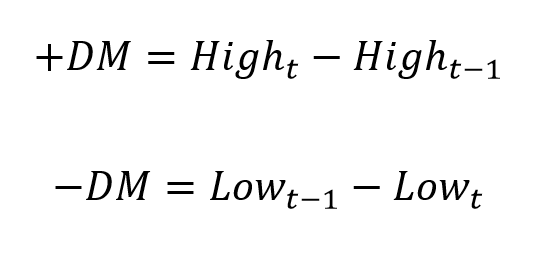Figure 1.2

This can be represented graphically (See Figure 1.3).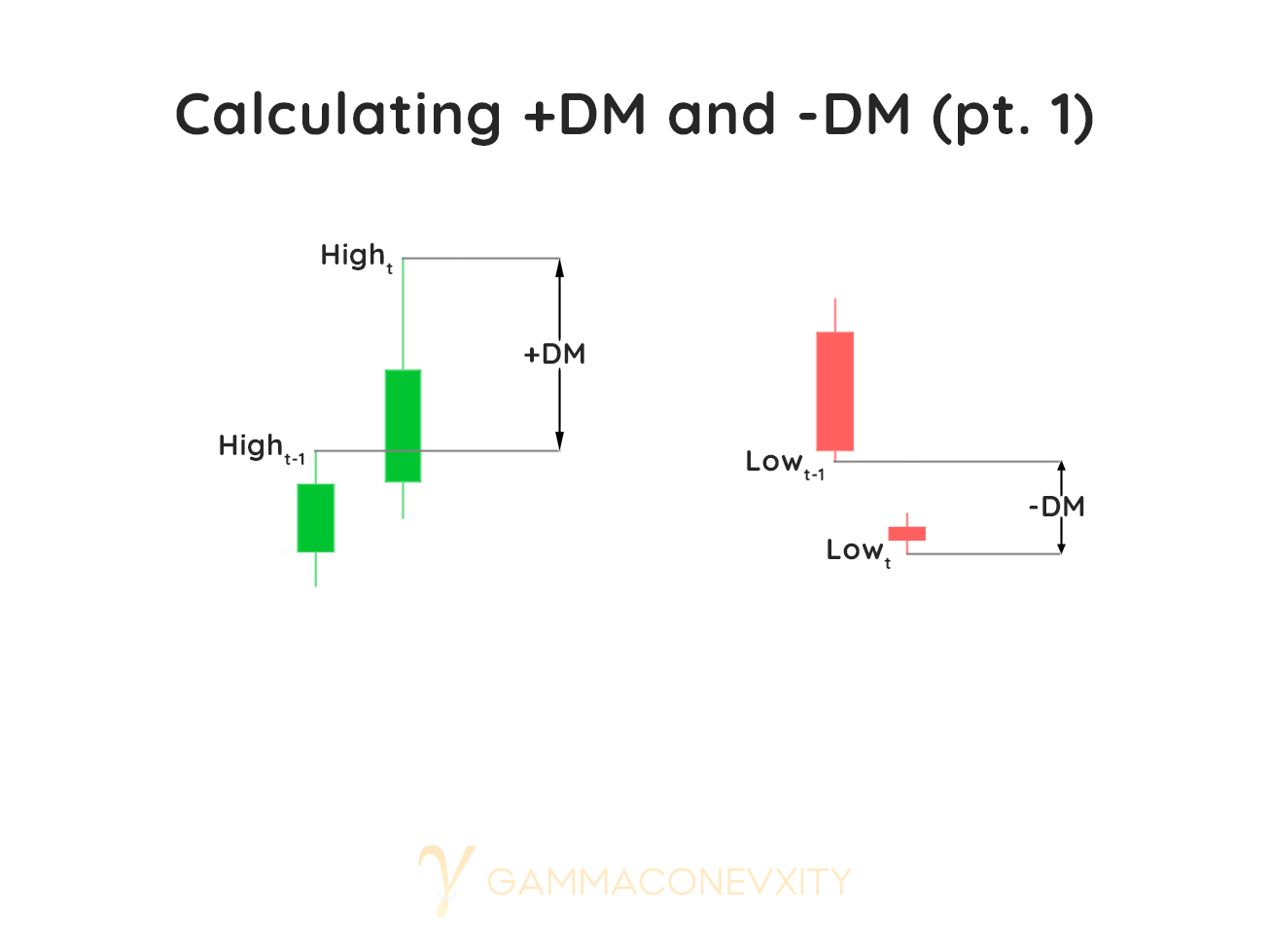Figure 1.3

Because both +DM and –DM can be calculated for every two consecutive candles, and because the goal of ADX is to find the highest magnitude trend, the lesser of the two values is set to zero. Directional movement outcomes are binary and must be either up or down. However, what would happen on an inside day, where the current period’s range is within the bounds of the previous candle? Or a neutral day, where the current candle the same range as the previous one? (See Figure 1.4)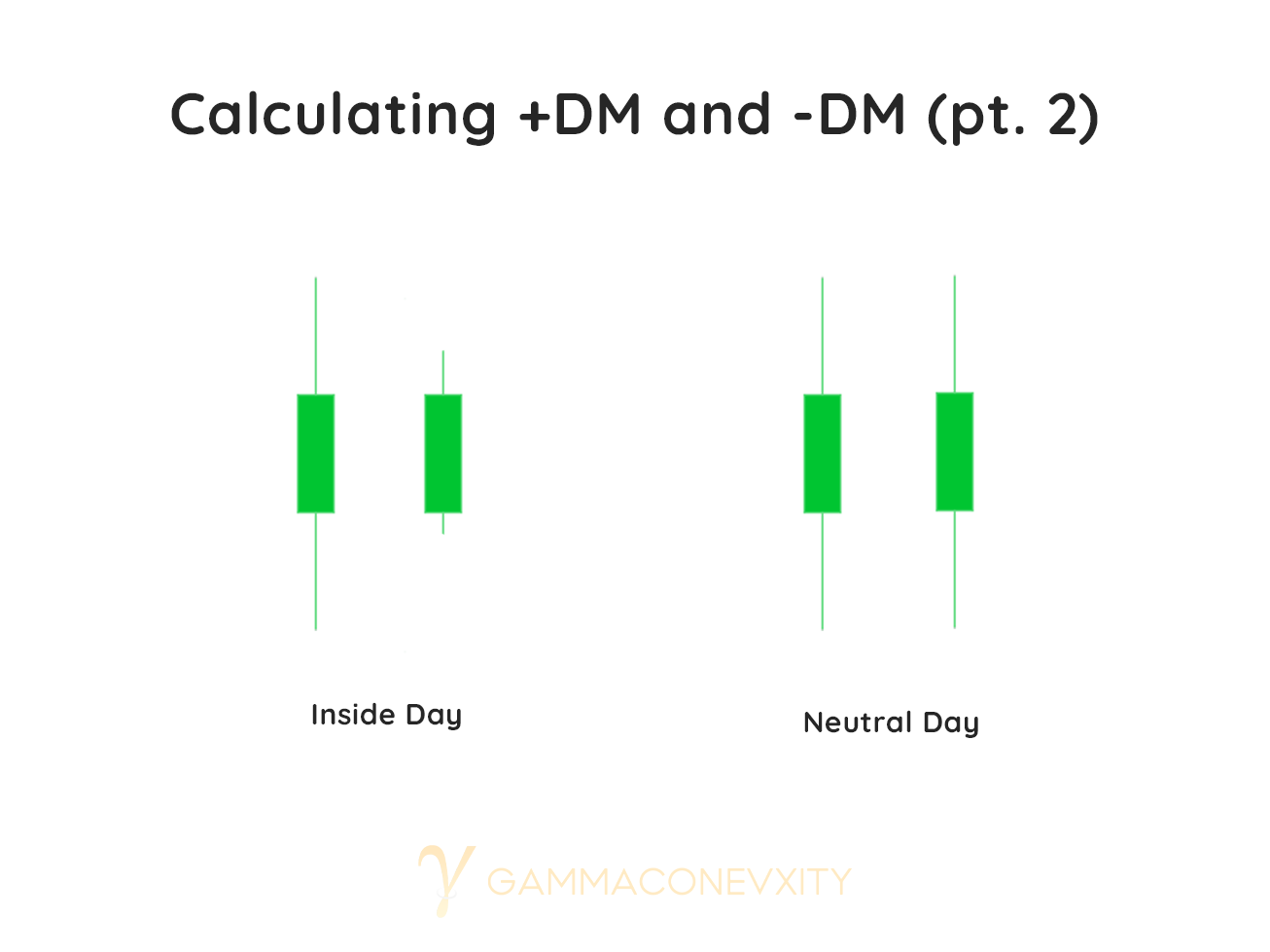Figure 1.4

On inside days, both +DM and –DM are negative, and both get set to zero because directional movement did not occur. On a neutral day, both +DM and –DM are already set to zero, so no modification to the original formula is needed. Factoring in these additional attributes, the final calculations for +DM and –DM is as follows (See Figure 1.5).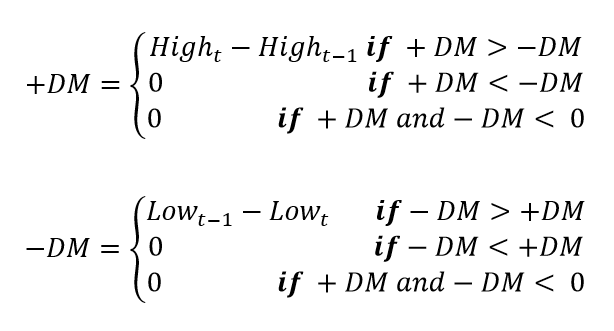Figure 1.5

2) True Range (TR), unlike +DM and –DM, is not a measure of directional movement but rather a measure of the volatility of price movement. It is calculated (See Figure 1.6) by finding the greatest of three measurements:

1. Current period’s high less the previous low
2. The absolute value of the current period’s high less the previous close
3. The absolute value of the current period’s low less the previous close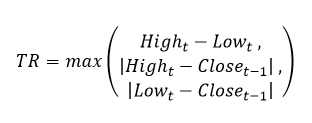Figure 1.6

This can be represented graphically (See Figure 1.7)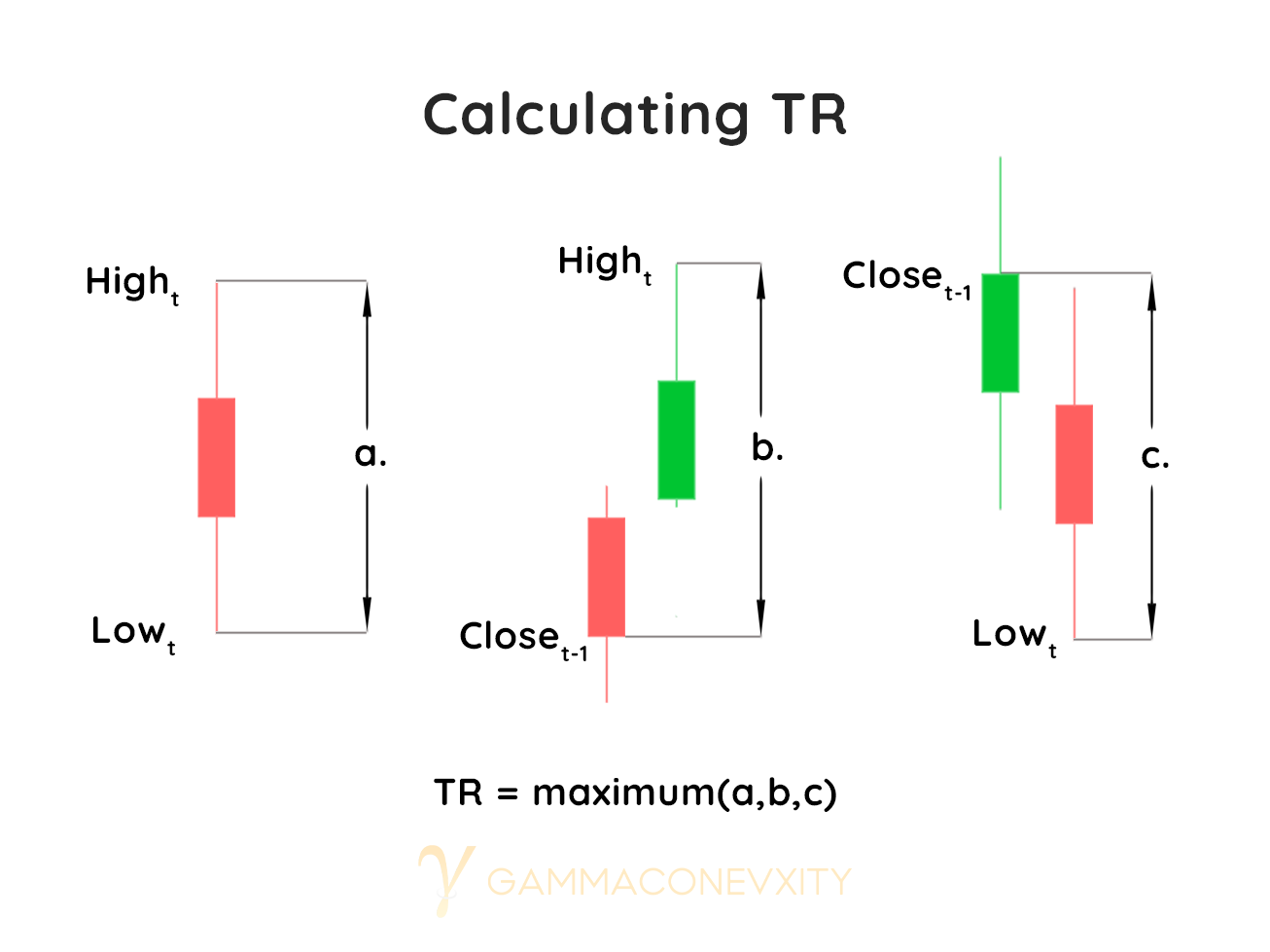Figure 1.7

3) The Positive Directional Indicator (+DI) and Negative Directional Indicator (-DI) are a combination of Directional Movement and True Range. The calculation is found simply by dividing +DM or –DM by the TR (See Figure 1.8).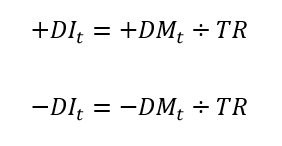Figure 1.8

The purpose of this calculation is to make the directional move of a given security relative to the trading range it was bound to at given time. Calculating the directional move of a security in isolation would be meaningless, as it could not be compared against other securities in a standardized manner. However, the equations above need a modification. They only calculate the +DI and -DI for a single period which is not implementable in practice. The current equations produce data with too much noise; if our team wished to create portfolio weights based on the equations in their current form, a single day’s outsized move would make an investment far more attractive than it truly is. To resolve this, the equations require rolling averages of both Directional Movement and True Range in order to smooth the data and generate reliable signals (See Figure 1.9). Wilder recommends a rolling lookback period of 14-days because it is an “average half-cycle period” (Wilder 38). By applying this period in the formulas below,  and  would be the average of the last 14 DM and TR values.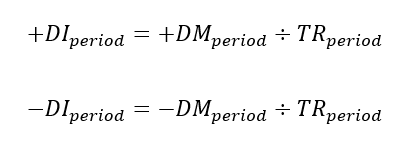Figure 1.9

Finally, we can calculate the Directional Movement Index (DX) by taking 100 times absolute value of  less the  divided by the sum of  and  (See Figure 1.10).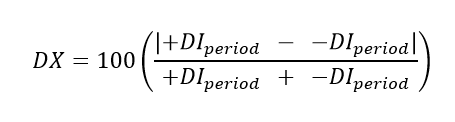Figure 1.10

The Average Directional Movement Index (ADX) is merely the  days rolling average of DX (See Figure 1.11)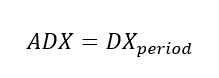Figure 1.11

Implementation:

To retrieve the necessary OHLC time-series data from Alphien, we made use of the Pandas data science library and stored all the data into Pandas DataFrames. What makes Pandas DataFrames special compared to other tabular data structures is that they are “two-dimensional, size-mutable, potentially heterogeneous tabular data,” perfect for a heterogeneous data set with many ticker names, price values, and timestamps (Pandas Development Team). Only HLC price data (no Open) was required to calculate ADX.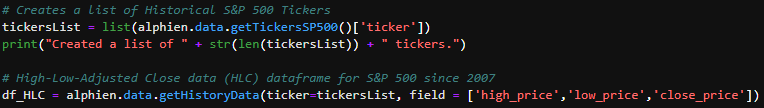Building the High-Low-Close DataFrame

Our implementation for each of the three ADX subcomponents is as follows:

1) Positive Directional Movement (+DM) and Negative Directional Movement (-DM)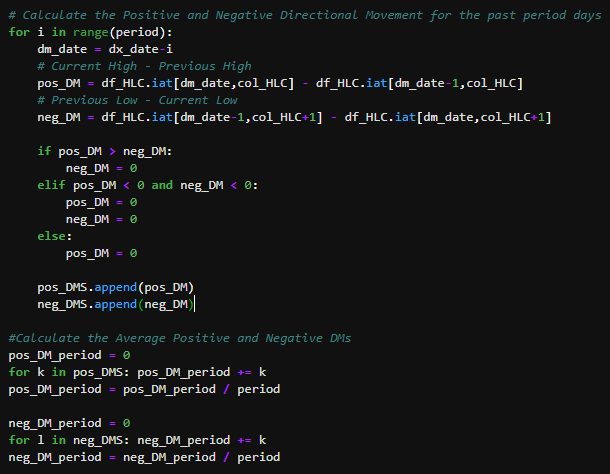Calculating +DM and -DM

2) True Range (TR)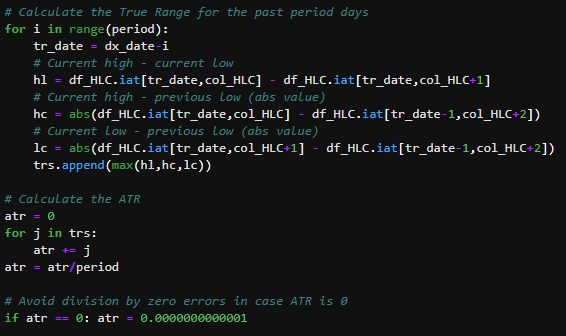Calculating TR

3) Positive Directional Indicator (+DI) and Negative Directional Indicator (-DI)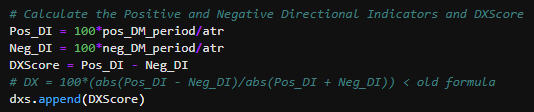Calculating +DI and -DI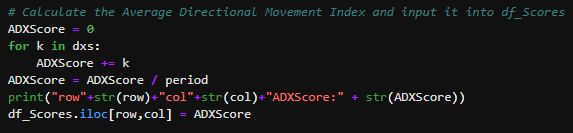As you may have noticed, compared to Wilder’s original calculations, our implementation of ADX deviated slightly in the calculation of DX and ADX itself. Instead of using the original formula for calculating DX, we removed the direction-neutralizing aspect of the formula, so instead of calculating DX, we calculated a new value called DXScore by simply subtracting -DI from +DI for the given period (See Figure 1.12).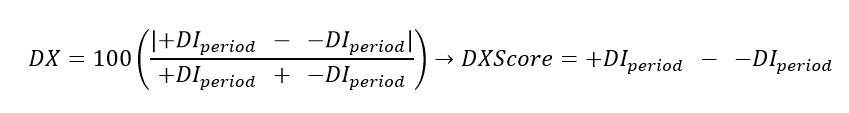Figure 1.12

The purpose of these modifications was to ensure that our portfolio took long-only (as per competition requirements) positions in S&P 500 companies with the strongest positively trending movement. Our portfolio would thus ignore companies with strong negative trends. ADX was simply renamed to ADXScore (See Figure 1.13), and the top 50 companies with highest ADXScore values were given an equal-weighted allocation in our portfolio.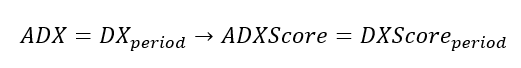Figure 1.13

Results

After conducting backtests (which took quite a while!) with varying rebalancing frequencies and rolling lookback periods, we obtained surprisingly good results and consistently outperformed the S&P 500 equal-weighted benchmark on every backtest. Our best backtest result came from a rebalancing frequency of every 30 days and a lookback period of 14 days. In this test, we had annualized returns of 20.42% (translating to 526.11% total returns), a Sharpe Ratio of 0.76, and a maximum drawdown of -52.62%. Our results stand to show that trend-following can be a legitimate strategy with recent historical outperformance relative to major indices—maybe the old trader’s adage “the trend is your friend” carries more weight than granted.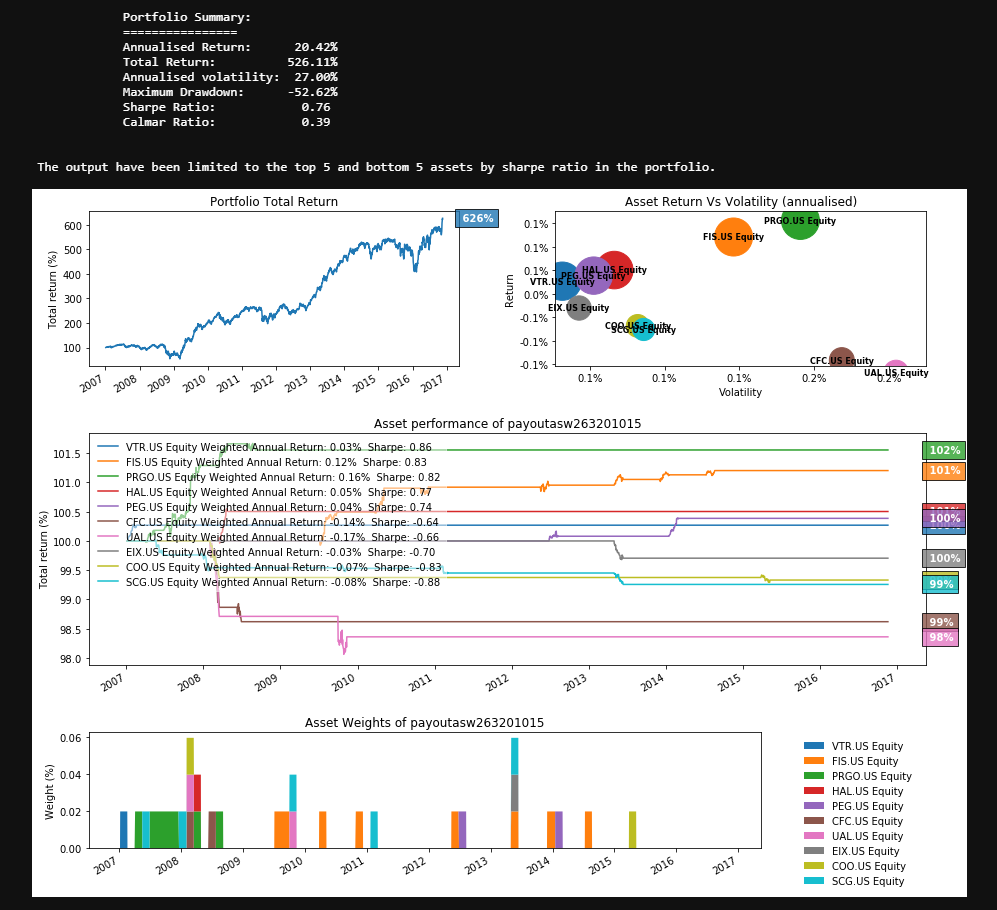Top Backtest Result

Reflections

Despite our strong portfolio returns, we did not end up making it to the final round of the 2020 UBS Quant Hackathon. While our portfolio outperformed in terms of pure return, the other aspects used to judge our strategy such as robustness or diversification were lacking. Our portfolio allocations were based on a single metric: strong upwards momentum. Despite this, the low granularity and frequency of the data (non-intraday, non-tick) made a trend-following algorithm relatively appropriate for the given task. Perhaps our strategy could have been made more robust by combining trend-following techniques with supervised learning regressions. With this added tool, our algorithm could filter correlative (w.r.t. the S&P 500) returning stocks and non-correlative returning stocks and choose the top 50 strongest-trending, lowest return-correlation stock picks. Hypothetically, this would decrease our maximum drawdown, increase our Sharpe Ratio, and improve our diversification.

Overall, I am very happy with just having been a participant of this competition. It was a wonderful learning experience on systemic trading, data science, and working with a team virtually. Our experience with this hackathon has inspired me to start Cornell’s first quantitative finance organization, so that students from any academic backgrounds can become quants! Hopefully, in next year’s hackathon, we will build a much more robust model (because we’ll finally know what we’re doing J) and make it to the finals.

Pandas Development Team. "pandas.DataFrame." 2021,

https://pandas.pydata.org/pandas-docs/stable/reference/api/pandas.DataFrame.html

Wilder, J. Welles. New concepts in technical trading systems. Trend Research, 1978.